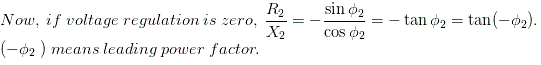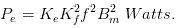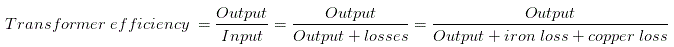# MCQs on Electrical Machines

##### Page 100 of 114. Go to page 1 2 3 4 5 6 7 8 9 10 11 12 13 14 15 16 17 18 19 20 21 22 23 24 25 26 27 28 29 30 31 32 33 34 35 36 37 38 39 40 41 42 43 44 45 46 47 48 49 50 51 52 53 54 55 56 57 58 59 60 61 62 63 64 65 66 67 68 69 70 71 72 73 74 75 76 77 78 79 80 81 82 83 84 85 86 87 88 89 90 91 92 93 94 95 96 97 98 99 100 101 102 103 104 105 106 107 108 109 110 111 112 113 114
01․ The primary and secondary windings of a transformer are

Whenever alternating voltage is applied to the primary of a electrical transformer, there will be a alternating flux through the core of transformer. As this flux will link with secondary winding there will be an induced emf in the secondary winding. The phenomenon of inducing voltage by changing flux is called electrical induction, hence it can be concluded that the windings of a transformer are linked inductively.

02․ At what power factor, the voltage regulation of a transformer can be zero ?
Lagging power factor.
Unity power factor.
Zero power factor.03․ In a three phase star - delta transformer, what is the angle difference between primary and secondary phase voltages?
Delta side lags by -30°.
Star side lags by -30°.

This is a vector group and has + 30° displacement. Therefore, delta side leads by + 30°.

04․ What will be the eddy current loss if the supply frequency of a transformer becomes double?
Eight times.
Four times.
Doubled.
Remains same.

Eddy Current loss in transformer is denoted as,Where, Ke = eddy current constant, Kf = form factor. Hence apparently, the eddy current loss Pe ∝ f2, but for any given voltage, if f decreases, Bm increases correspondingly and if f increases Bm decreases correspondingly. Hence the eddy current loss Pe at any given voltage, is independent of frequency.

05․ The efficiency of a transformer is mainly dependent on
core losses.
copper losses.
stray losses.
dielectric losses.Iron loss is caused by the alternating flux in the core and consists of hysteresis and eddy current losses. These losses occur in the core and yoke due to alternating flux. Copper losses occur in the primary and secondary windings due to their resistances when the transformer is loaded. Iron losses ( core losses ) are independent of load, and therefore are called constant losses. Generally at maximum efficiency the core loss of transformer is equal to copper loss. Again the transformers are so designed that maximum efficiency of transformer reaches at full load current. So for all other load copper loss is less than core loss hence core loss dose have maximum value among all other losses in a power transformer and that is why the efficiency of a transformer mainly depends upon core losses.

06․ If supply frequency of a transformer decreases,
both eddy current and hysteresis losses will increase.
eddy current loss will increase but hysteresis loss
eddy current loss remains same and hysteresis losses will increase.
eddy current loss will decrease but hysteresis loss
The total core loss Pc in terms of voltage and frequency is Pc = hysteresis loss + eddy current loss = KhVxf 1 - x + KeV2. Hence, eddy current is independent of frequency and hysteresis loss is inversely varies with frequency.

07․ Transformers operating in parallel will share the load depending upon their
leakage reactance.
rating.
efficiency.
percentage impedance.

The load sharing by the transformers must be proportionate with their MVA rating.

08․ No load primary current in a transformer
lags behind applied voltage somewhat less than 90Ã
lags behind applied voltage by exactly 90Ã‚&am
leads the applied voltage by exactly 90Ã‚&
leads the applied voltage somewhat less than 90Ã&

As the winding of the transformer is inductive the magnetizing current in it lags behind the primary applied voltage by exactly 90° but there is a core loss component which will be in phase with applied voltage. The no load current is the vector sum of these two currents hence this must lag behind applied voltage somewhat less than 90°.

09․ Which of the following is minimized by laminating the core of a transformer?
Hysteresis loss.
Eddy current loss.
Copper loss.
Stray loss.

By laminating the core of transformer we make the eddy current circulating path narrow, means increasing the resistance of eddy current and proportionately reducing the eddy current. Although the resistance of eddy current path Re increases, but as the eddy current Ie reduces, the overall eddy current loss Ie2Re is reduced.

10․ Breather is provided in a transformer to
absorb moisture of air during breathing.
provide cold air in transformer.
absorb moisture from air entering in transformer.
filter the transformer oil.

Whenever transformer oil becomes hot it expands in volume, the air on the top of the oil in conservator tank will come out through the breather and when oil becomes cold the atmospheric air enters in the transformer through this breather. This is called breathing of transformer. Silica gel is provided in the breather pot which absorbs moisture hence air becomes dry during passing through the breather. So breather is provided in a transformer to absorb moisture from air entering in transformer as well as provide breathing to the transformer.

<<<9899100101102>>>